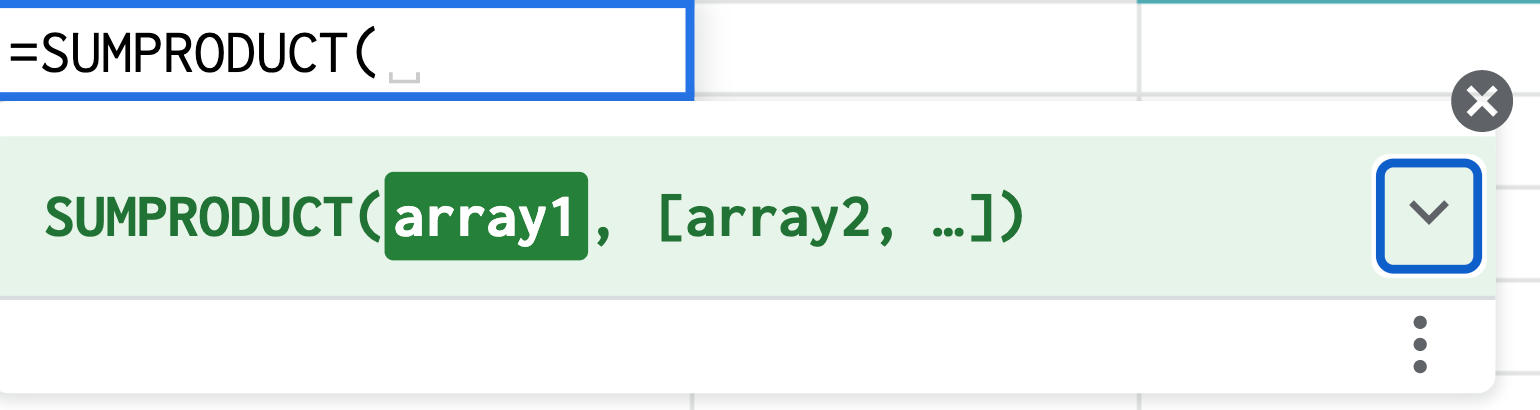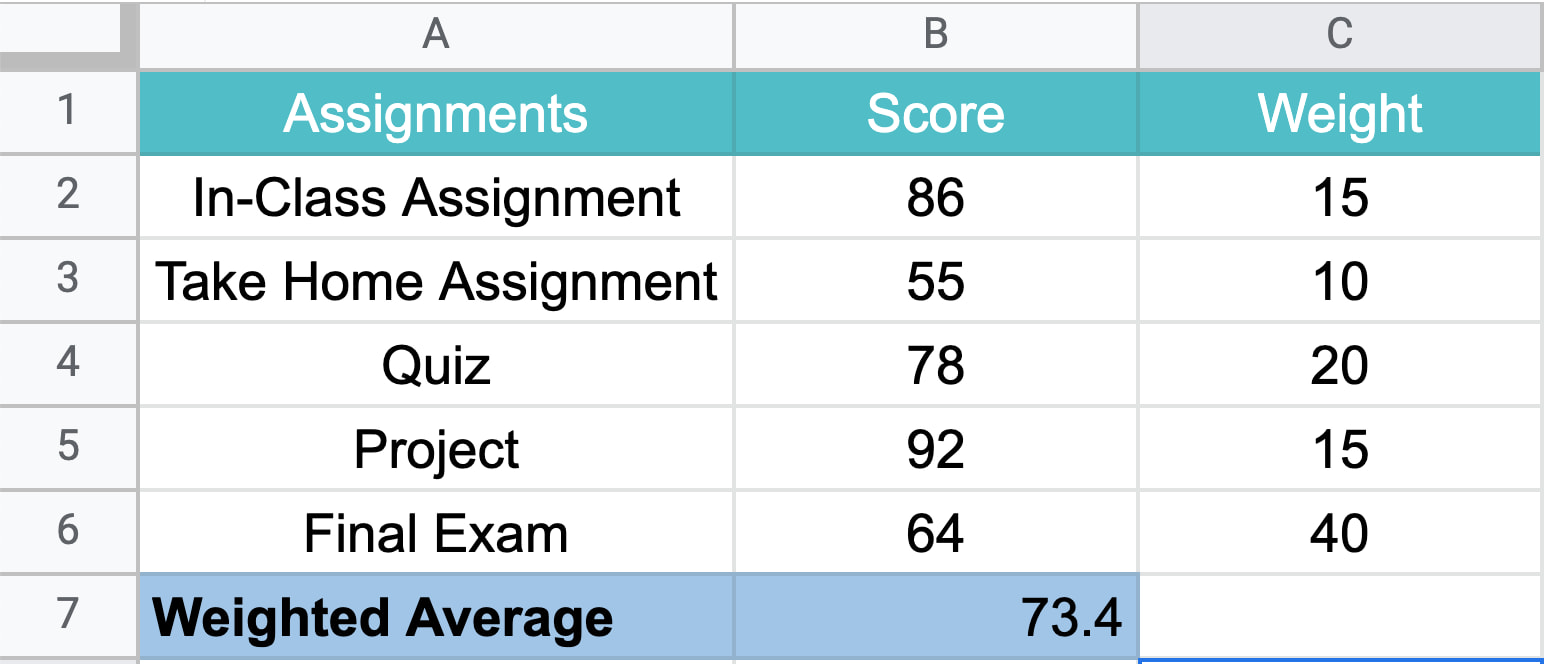# How to Use the Weighted Average Formula in Excel

How to Use the Weighted Average Formula in Excel

When you’re calculating the average for a set of values, you’re generally working with values that have the same weight and importance.

But what happens if some values weigh more than others? This is where the weighted average formula comes in.

In this article, we will break down how to use this formula in Excel, plus provide some examples.

## How to Calculate Weighted Average in ExcelTo calculate the weighted average in Excel, you must use the SUMPRODUCT and SUM functions using the following formula:

=SUMPRODUCT(X:X,X:X)/SUM(X:X)

This formula works by multiplying each value by its weight and combining the values. Then, you divide the SUMPRODUCT but the sum of the weights for your weighted average.

Still confused? Let’s go over the steps in the next section.

## Using SUMPRODUCT to Calculate Weighted Average in Excel

### 3. Click enter to get your results.## How to Find Weighted Moving Averages in Excel

A weighted moving average is a technique used to keep the time period of the average the same as you add new data or give more weight to certain time periods. This can allow you to identify trends and patterns more easily.

For instance, say you have the number of views your website got in the last five days, you can easily determine the average views in a five-day period.

Now, say the next week, I ask for the five-day average, you would use data from the last five days, not the original five days from the previous week.

As such, you’re still relying on the same time period but updating the data to generate the moving average.

For a weighted moving average, you give more weight to certain time periods than others. You may say that day 5 weights 60% with the remaining percentages decreasing by day.

As such, you’ll need to manually calculate this formula.

WMA = [value 1 x (weight)] + [value 2 x (weight)] + [value 3 x (weight)] + [value 4 x (weight)]

Once you get the hang of it, using the weighted average formula is easy. All it takes is a little practice.Go to Source